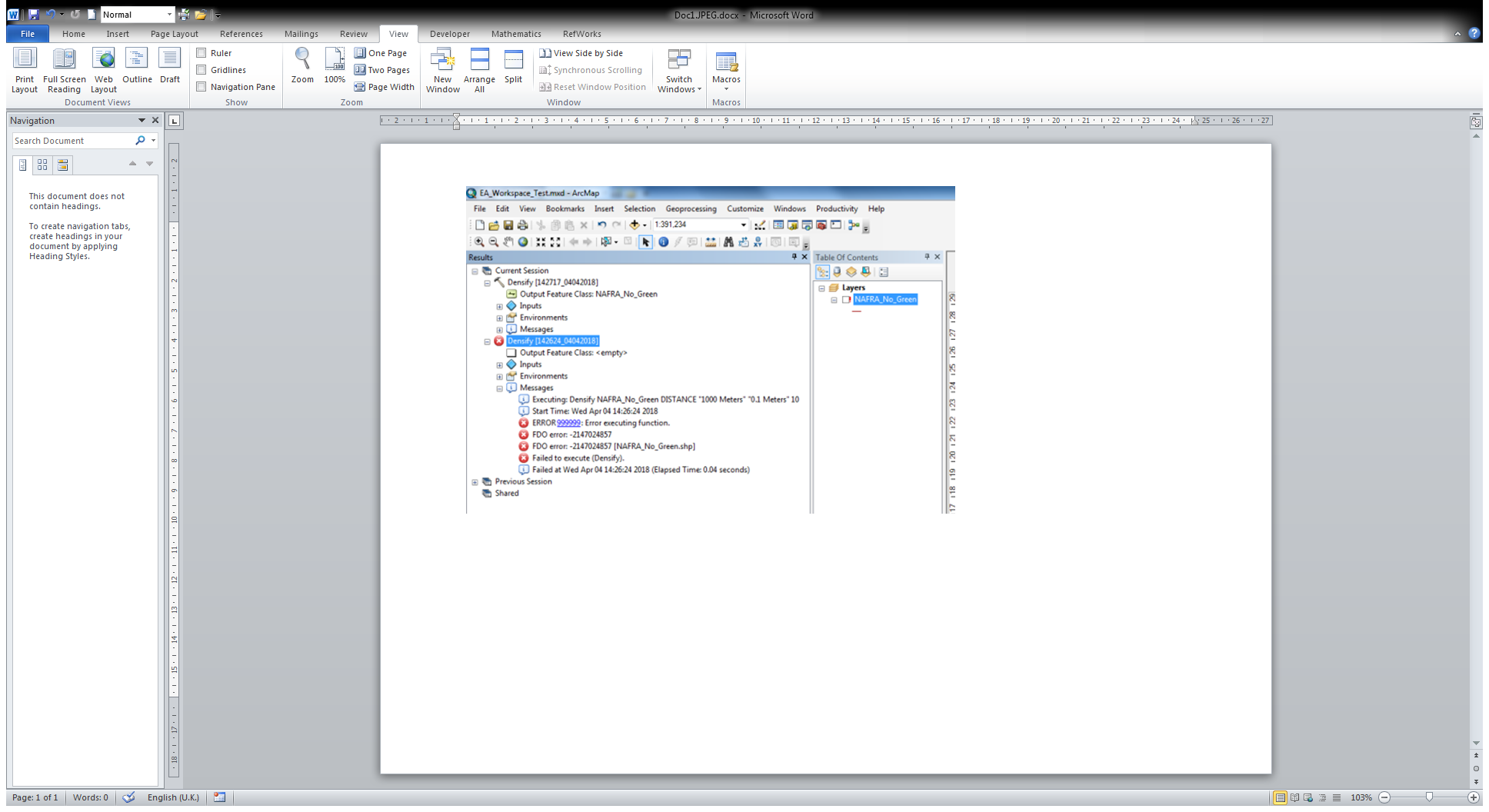# Splitting polylines

988
9
04-04-2018 06:11 AMNew Contributor II

Hi there.

I have a polyline layer with a few thousand lines within. I want to split these lines into segments but cannot find a way of doing so.

I tried running 'Generate points along lines' and then using 'Split lines by points', however this method is splitting my lines into too many segments. I think when I set the search radius the tool is using points that are on the other lines in the layer to split; but if I lower the search radius the tool won't pick up all the points along the line to split it correctly.

I have seen there's a technique to doing this with the editor toolbar, however I need to generate the python script for the tool, and from what I've seen there is no script for this method of splitting.

I'm using ArcMap 10.5.1

Anyone any ideas? Thanks.

1 Solution

Accepted SolutionsbyEsri Esteemed Contributor

Here is a snippet that could work:

``````def main():
import arcpy
import os

# input parameters
fc_in = r'C:\GeoNet\CoastFlooding\test.gdb\coast'  # path to input featureclass
fc_out = r'C:\GeoNet\CoastFlooding\test.gdb\coast_segments3'  # path to output featureclass
segment_length = 1000.0  # length of each segment, change this!

# create empty output featureclass with same template for fields
ws, fc_name = os.path.split(fc_out)
sr = arcpy.Describe(fc_in).spatialReference
arcpy.CreateFeatureclass_management(ws, fc_name, "POLYLINE", fc_in, None, None, sr)

# get all fields
flds = GetAllField(fc_in)

# create the features
cnt_in = 0
cnt_out = 0
with arcpy.da.InsertCursor(fc_out, flds) as curs_out:
with arcpy.da.SearchCursor(fc_in, flds) as curs_in:
for row_in in curs_in:
cnt_in += 1
polyline_in = row_in
from_dist = 0
if cnt_in % 100 == 0:
print("Processing line: {}, generated {} segments".format(cnt_in, cnt_out))
while from_dist <= polyline_in.length:
cnt_out += 1
to_dist = from_dist + segment_length
segment = polyline_in.segmentAlongLine(from_dist, to_dist, False)
lst_out = list(row_in)
lst_out = segment
row_out = tuple(lst_out)
curs_out.insertRow(row_out)
from_dist += segment_length

def GetAllField(fc):
exclude_fields = []
fld_names =[fld.name for fld in arcpy.ListFields(fc)]

try:
exclude_fields.append(arcpy.Describe(fc).AreaFieldName)
except:
pass
try:
exclude_fields.append(arcpy.Describe(fc).LengthFieldName)
except:
pass
try:
exclude_fields.append(arcpy.Describe(fc).ShapeFieldName)
except:
pass

for exclude_field in exclude_fields:
if exclude_field in fld_names:
fld_names.remove(exclude_field)

# include shape field at 1st position
fld_names.insert(0, 'SHAPE@')
return fld_names

if __name__ == '__main__':
main()‍‍‍‍‍‍‍‍‍‍‍‍‍‍‍‍‍‍‍‍‍‍‍‍‍‍‍‍‍‍‍‍‍‍‍‍‍‍‍‍‍‍‍‍‍‍‍‍‍‍‍‍‍‍‍‍‍‍‍‍‍‍‍‍‍‍``````

The resulting attribute tables are like this:9 RepliesbyEsri Esteemed Contributor

Can you explain what logic you want to apply to split each line into segments? Does this have to happen at each vert ice or every # distance along the line? Should  the attributes remain, is there any "split policy" you want to apply in the process?New Contributor II

I want the line to be split at every # distance along the line, whilst keeping the attributes of the original line. These are really the only criteria that is important to me.byMVP Esteemed ContributorNew Contributor II

Ah, I tried using densify and it broke my data layer. I'll have a play around with that tool and see if I can get that to work.byEsri Esteemed Contributor

That is possible using some Python code and the Polyline::segmentAlongLine So there is no (numeric) attribute that should be modified representing the percentage of the segment? Does the line have Z and/or M values?New Contributor II

I just want each segment (let's say each line is split into quarters) to retain all the attributes of the original line, whilst also having some form of identification (the FID will suffice if one is generated). I don't mind if this alters the original layer or creates a new one.

There are no Z or M values.byEsri Esteemed Contributor

Here is a snippet that could work:

``````def main():
import arcpy
import os

# input parameters
fc_in = r'C:\GeoNet\CoastFlooding\test.gdb\coast'  # path to input featureclass
fc_out = r'C:\GeoNet\CoastFlooding\test.gdb\coast_segments3'  # path to output featureclass
segment_length = 1000.0  # length of each segment, change this!

# create empty output featureclass with same template for fields
ws, fc_name = os.path.split(fc_out)
sr = arcpy.Describe(fc_in).spatialReference
arcpy.CreateFeatureclass_management(ws, fc_name, "POLYLINE", fc_in, None, None, sr)

# get all fields
flds = GetAllField(fc_in)

# create the features
cnt_in = 0
cnt_out = 0
with arcpy.da.InsertCursor(fc_out, flds) as curs_out:
with arcpy.da.SearchCursor(fc_in, flds) as curs_in:
for row_in in curs_in:
cnt_in += 1
polyline_in = row_in
from_dist = 0
if cnt_in % 100 == 0:
print("Processing line: {}, generated {} segments".format(cnt_in, cnt_out))
while from_dist <= polyline_in.length:
cnt_out += 1
to_dist = from_dist + segment_length
segment = polyline_in.segmentAlongLine(from_dist, to_dist, False)
lst_out = list(row_in)
lst_out = segment
row_out = tuple(lst_out)
curs_out.insertRow(row_out)
from_dist += segment_length

def GetAllField(fc):
exclude_fields = []
fld_names =[fld.name for fld in arcpy.ListFields(fc)]

try:
exclude_fields.append(arcpy.Describe(fc).AreaFieldName)
except:
pass
try:
exclude_fields.append(arcpy.Describe(fc).LengthFieldName)
except:
pass
try:
exclude_fields.append(arcpy.Describe(fc).ShapeFieldName)
except:
pass

for exclude_field in exclude_fields:
if exclude_field in fld_names:
fld_names.remove(exclude_field)

# include shape field at 1st position
fld_names.insert(0, 'SHAPE@')
return fld_names

if __name__ == '__main__':
main()‍‍‍‍‍‍‍‍‍‍‍‍‍‍‍‍‍‍‍‍‍‍‍‍‍‍‍‍‍‍‍‍‍‍‍‍‍‍‍‍‍‍‍‍‍‍‍‍‍‍‍‍‍‍‍‍‍‍‍‍‍‍‍‍‍‍``````

The resulting attribute tables are like this:New Contributor II

Many thanks friend. You were a big help.byEsri Esteemed Contributor

Hi Chris Marsh , did the code work for you? If not, please post back any changes that may be required. If it did work for you, could you mark the answer as correct?